# 1 7 Scientific Notation Scientific Notation At times

• Slides: 10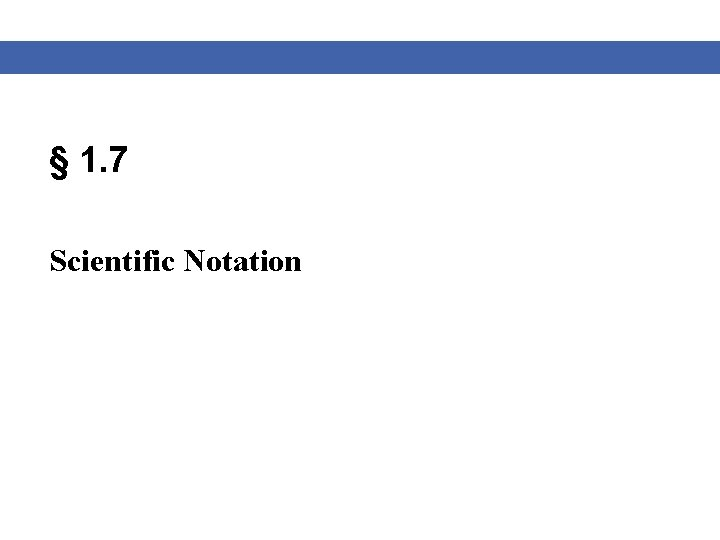§ 1. 7 Scientific NotationScientific Notation At times you may find it necessary to work with really large numbers or alternately really small numbers. In this section, you will learn how to write these often cumbersome numbers in scientific notation. A number is written in scientific notation when it is expressed as the product of a number between one and ten and some power of ten. Blitzer, Algebra for College Students, 6 e – Slide #2 Section 1. 7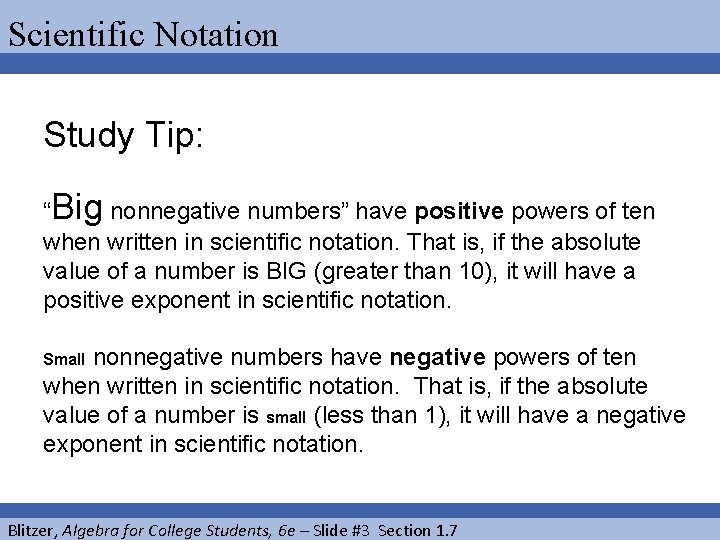Scientific Notation Study Tip: “Big nonnegative numbers” have positive powers of ten when written in scientific notation. That is, if the absolute value of a number is BIG (greater than 10), it will have a positive exponent in scientific notation. nonnegative numbers have negative powers of ten when written in scientific notation. That is, if the absolute value of a number is small (less than 1), it will have a negative exponent in scientific notation. Small Blitzer, Algebra for College Students, 6 e – Slide #3 Section 1. 7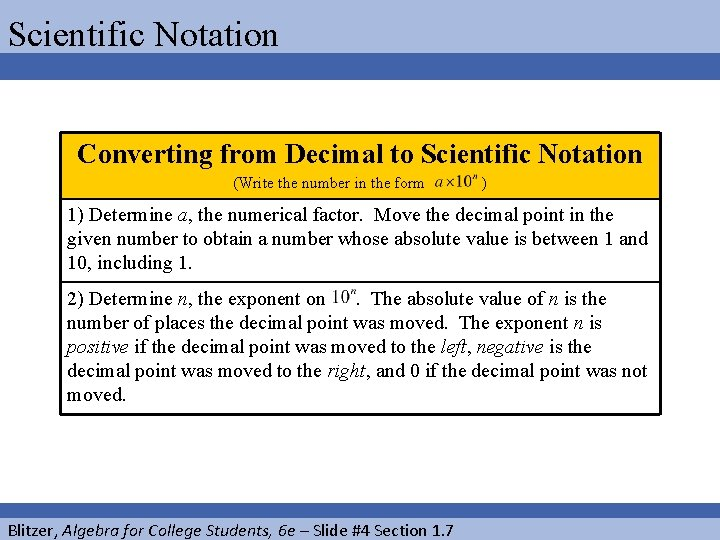Scientific Notation Converting from Decimal to Scientific Notation (Write the number in the form ) 1) Determine a, the numerical factor. Move the decimal point in the given number to obtain a number whose absolute value is between 1 and 10, including 1. 2) Determine n, the exponent on. The absolute value of n is the number of places the decimal point was moved. The exponent n is positive if the decimal point was moved to the left, negative is the decimal point was moved to the right, and 0 if the decimal point was not moved. Blitzer, Algebra for College Students, 6 e – Slide #4 Section 1. 7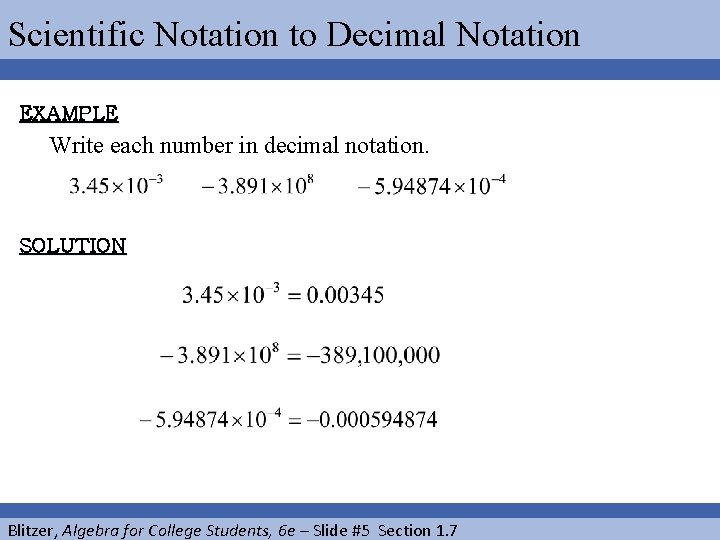Scientific Notation to Decimal Notation EXAMPLE Write each number in decimal notation. SOLUTION Blitzer, Algebra for College Students, 6 e – Slide #5 Section 1. 7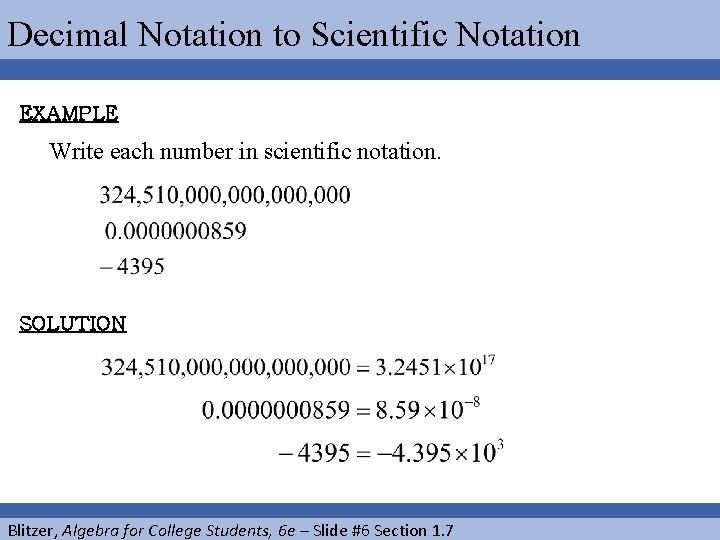Decimal Notation to Scientific Notation EXAMPLE Write each number in scientific notation. SOLUTION Blitzer, Algebra for College Students, 6 e – Slide #6 Section 1. 7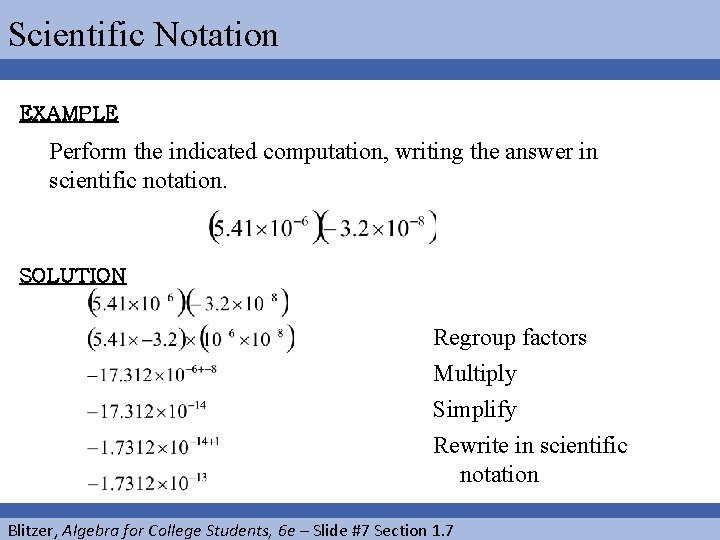Scientific Notation EXAMPLE Perform the indicated computation, writing the answer in scientific notation. SOLUTION Regroup factors Multiply Simplify Rewrite in scientific notation Blitzer, Algebra for College Students, 6 e – Slide #7 Section 1. 7Scientific Notation EXAMPLE Perform the indicated computation, writing the answer in scientific notation. SOLUTION Group factors Divide Simplify Write in scientific notation Blitzer, Algebra for College Students, 6 e – Slide #8 Section 1. 7Scientific Notation EXAMPLE The area of Alaska is approximately acres. The state was purchased in 1867 from Russia for \$7. 2 million. What price per acre, to the nearest cent, did the United States pay Russia? SOLUTION We will first write 7. 2 million in scientific notation. It is: Now we can answer the question. Blitzer, Algebra for College Students, 6 e – Slide #9 Section 1. 7Scientific Notation CONTINUED Divide ‘dollar amount’ by ‘acreage’ Simplify Divide Subtract Therefore, since dollars/acre equals \$0. 0197/acre, then the price per acre for Alaska was approximately 2 cents per acre. WOW!!! Blitzer, Algebra for College Students, 6 e – Slide #10 Section 1. 7